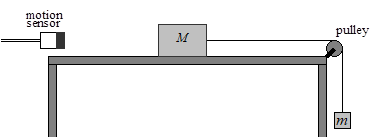# Coefficient of Friction

The friction force is the force exerted by a surface when an object moves across it - or makes an effort to move across it. The coefficient of friction is the ratio of the frictional force resisting the motion of two surfaces in contact. This is explored in this lab using a wooden block pulled across a wooden board.The above illustration shows the set-up of the epxeriment. The wooden block of mass M is accelerated along the wooden board by use of a light string attached to the block passing over a pulley and a mass m hangs from the end of the string. When m is released the block accelerates across the board.

The acceleration of the block is given by

a=(mg-(μ_k*M*g))/(m+M)

where μ_k is the coefficient of kinetic friction between the two surfaces.  If the table surface is reasonably uniform, all the terms on the right hand side of equation are constant and hence the block should move with constant acceleration.

In the diagram you can see a motion sensor. The motion sensor and its associated software (Loggerpro) can be used to record and display the position (x) of the block as a function of time (t) while the block is in motion.

If you want to explore the concepts of friction and force try you hand with these online labs / simulations:

Friction

Force Required to Move a Wooden Block

Friction Lab

## Department of Physics

Roinn na Fisice

Room 213 (Physics Office), 2nd floor, Kane Science Building, University College Cork, Ireland.,

Top